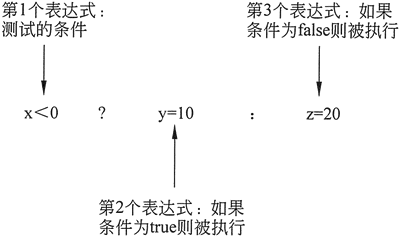## C++ ?:条件运算符(三目运算符)用法详解

• 内容
• 评论
• 相关

x < 0 ? y = 10 : z = 20;

```if (x < 0)
y = 10；
else
z = 20;```

(x < 0) ? (y = 10) : (Z = 20);0条评论